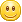# 使用PHP做网页采集实例过程总结

```\$conn_1=pg_connect("host=xxx.xxx.xxx.xxx port=5432 dbname=mydb1 user=postgres password=xxxxxx") ;
\$conn_2=pg_connect("host=xxx.xxx.xxx.xxx port=5432 dbname=mydb2 user=postgres password=xxxxxx") ;
```

```
//获取网页源码
//\$url='https://zhangnq.com/' ;
\$str = file_get_contents(\$url);
//使用preg_match和正则表达式取出编码
\$wcharset = preg_match("/<meta.+?charset=[^\w]?([-\w]+)/i",\$str,\$temp) ? strtolower(\$temp):"" ;
//编码转换
if(\$wcharset){
\$str=iconv("\$wcharset", "UTF-8", \$str) ;
}

```

```
\$pat = "/<a(.*?)href=\"(\$preg)\"(.*?)>(.*?)<\/a>/is";
preg_match_all(\$pat, \$str, \$m);
\$cnt=count(\$m) ;
for(\$i=0;\$i<\$cnt;\$i++){
if(strip_tags(\$m[\$i])){
\$url=strip_tags(\$m[\$i]) ;
\$url=\$m[\$i] ;
}
if(strip_tags(\$m[\$i])){
\$title=strip_tags(\$m[\$i]) ;
}
else{
\$title="There's Something Errors!" ;
}
//编写代码，对title和url进行入库操作。
}
}

```

Comment (2)
1.小行 　( 2014.02.21 12:54 ) : #-9

赞一个，真心不错•章郎虫 　( 2014.02.21 15:25 ) :

谢谢，业余的，写的不好。